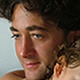# Blog

Learn about industry trends, news and how-tos from our product experts.

## Drawing 2D-01

const string dimLayerName = "Dimension", linetypeName = "DashDot", referenceLayerName = "Reference"; Plane vertical = new Plane(Point3D.Origin, Vector3D.AxisY, -1 * Vector3D.AxisX); // plane.XY; // adding different layers design1.LineTypes.Add(linetypeName, new float[] { 10, -2, 2, -2 }); design1.Layers.LineWeight = 2; design1.Layers.Add(new Layer(dimLayerName, Color.CornflowerBlue) { LineWeig…by Gianpiero Tovo | October 13, 2023 | Share

// main body arcs definitions Arc a1 = new Arc(Plane.XY, new Point3D(-20, -15), new Point3D(-22, 0), new Point3D(-20, 15), true); Arc a2 = new Arc(Plane.XY, new Point3D(-20, 15), new Point3D(0, 16.5), new Point3D(20, 15), true); Arc a3 = new Arc(Plane.XY, new Point3D(20, 15), new Point3D(22, 0), new Point3D(20, -15), true); Arc a4 = new Arc(Plane.XY, new Point3D(20, -15), new Point3D(0, -16.5), new Point3D(-20, -15), true); double filletRadius = 8; Cur…by Gianpiero Tovo | September 21, 2023 | Share

## Creating a BRep entity from scratch

In this article, we create a BRep entity by defining its vertices, edges, faces and shells. const double radius = 10, height = 20, centreToCentre = 20, offset = 3; Point3D[] vertices = new Brep.Vertex; vertices = new Brep.Vertex(0, -radius); vertices = new Brep.Vertex(centreToCentre, -radius); vertices = new Brep.Vertex(centreToCentre, +radius); vertices = new Brep.Vertex(0, +radius); vertic…by Alberto Bencivenni | July 28, 2023 | Share

## Modeling Practice Drawings 110

// Main polygon CompositeCurve cc1 = new CompositeCurve( new Line(-34, 0, 34, 0), new Line(34, 0, 34, 59.4), new Arc(Plane.XY, new Point2D(0, 59.4), 34, Utility.DegToRad(0), Utility.DegToRad(180)), new Line(-34, 59.4, -34, 0)); devDept.Eyeshot.Entities.Region reg1 = new devDept.Eyeshot.Entities.Region(cc1); Brep ext1 = reg1.ExtrudeAsBrep(new Interval(0, 26)); // Borders devDept.Eyeshot.Entities.Region reg2 = devD…by Paolo Bencivenni | July 24, 2023 | Share

## Modeling Practice Drawings 91

// Big slot devDept.Eyeshot.Entities.Region reg1 = devDept.Eyeshot.Entities.Region.CreateSlot(0, 0,22.5, 18, 0, true); Brep ext1 = reg1.ExtrudeAsBrep(new Interval(0, 4.4)); // Small slot devDept.Eyeshot.Entities.Region reg2 = devDept.Eyeshot.Entities.Region.CreateSlot(0, 0, 22.5, 7.6, 0, true); ext1.ExtrudeAdd(reg2, new Interval(0, 16)); devDept.Eyeshot.Entities.Region reg3 = devDept.Eyeshot.En…by Paolo Bencivenni | July 24, 2023 | Share

## Modeling Practice Drawings 69

At the time of writing, due to a singularity, we need to set the radius as 19 units instead of 20. // Sketch 1 var sk1 = new devDept.Geometry.ConstraintSolver.Sketch(); // Add origin point SketchPoint origin1 = sk1.AddOrigin(); // Lines var l1 = sk1.AddLine(-20, -60, -20, -100); var l2 = sk1.AddLine(-20, -100, 20, -100); var l3 = sk1.AddLine(20, -100, 20, -60); // Mid point var p1 = sk1.AddPoint(20, -100); sk1.AddConstraintMidP…by Paolo Bencivenni | July 20, 2023 | Share

## 3D-DP949

// Main polygon devDept.Eyeshot.Entities.Region cir1 = devDept.Eyeshot.Entities.Region.CreateCircle(Plane.YZ, 0, 76, 20); Line lR = UtilityEx.GetLinesTangentToCircleFromPoint((Circle)cir1.ContourList, new Point3D(0, -32, 20)); Line lL = UtilityEx.GetLinesTangentToCircleFromPoint((Circle)cir1.ContourList, new Point3D(0, 32, 20)); Arc arc = new Arc(Plane.YZ, new Point2D(0, 76), 20…by Paolo Bencivenni | July 12, 2023 | Share

## Modeling Practice Drawings 101

// Main polygon devDept.Eyeshot.Entities.Region reg1 = devDept.Eyeshot.Entities.Region.CreatePolygon(Plane.XZ, new Point2D[]{ new Point2D(0, 0), new Point2D(92, 0), new Point2D(92, 13), new Point2D(19,13), new Point2D(19, 129), new Point2D(6, 129), new Point2D(6, 113.5), new Point2D(10, 113.5), new Point2D(10, 100.5), new Point2D(6, 100.5), new Point2D(6, 13), new Point2D(0, 13) }); Brep ext1 = reg1.ExtrudeAsBrep(new…by Paolo Bencivenni | July 11, 2023 | Shareby Paolo Bencivenni | July 11, 2023 | Shareby Alberto Bencivenni | June 30, 2023 | Share